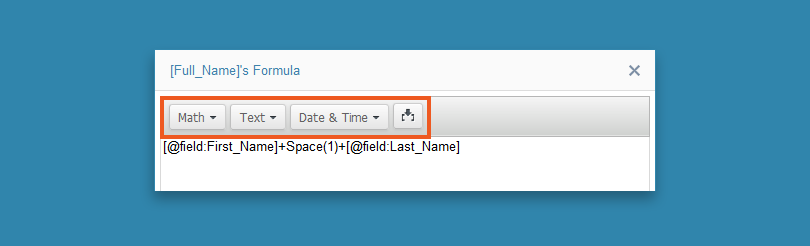Add a formula field that calculates a value based on other fields in the same table. In the formula field, you create an expression that uses values from fields, constants, or functions. For example, you can combine the first name and last name to create a single full name. When you have a table with product orders, you can calculate the total cost of each record based on its price and quantity. You can enter up to 10,000 characters in a single formula.

See or read how you can do it:

1. Create a new table or open an existing table in the design mode to add a field. For more information, see Modifying a Table’s Design.
2. In the first empty row, enter a name for the field and select the Formula data type.
3. On the right pane, in the Options section, click Edit.
4. In the Formula dialog box, enter your formula. You can also insert built-in functions or incorporate fields of the record from the drop-down lists. For example, [@field:First_Name]+Space(1)+[@field:Last_Name].5. To confirm that the formula is valid, click Verify Formula.

Note: The formula is not valid when it contains SQL subqueries, aggregate functions (SUM, AVERAGE), nondeterministic functions (GetUTCDate, Rand), or other formula fields.

6. Optional: To prevent duplicate values in the field, select the Unique checkbox.

Note: If the application user tries to insert or update a record that makes the calculated value of the formula field not unique, the table does not store the changes.

7. Click Apply to close the dialog box, then click Save.

You can now see the new Formula field in Datasheet view. The expression that you entered on the Table Design tab calculates the values for the Formula field.### Good to know

• To pass and receive the value of a Formula field as a parameter, use the following syntax [@InsertRecordFieldName]. For more information, see Parameters.
• To build complex conditional formulas in a Formula field, use the CASE expression with its CASE WHEN THEN ELSE construct in T-SQL. For more information, watch the video from the beginning of this article and see the official Microsoft documentation about Transact-SQL.
• To export and import Formula fields as a formula, use the Caspio XML format.
• To export and import Formula fields as a value, use the CSV, Excel, or MS Access formats.